## Monday, November 19, 2018

### Oracle Machine Learning notebooks

In this blog post I'll have a look at Oracle Machine Learning notebooks, some of the example notebooks and then how to create a new one.

Check out my previous blog posts on ADWC.

On entering Oracle Machine Learning on your ADWC service, you will get the following.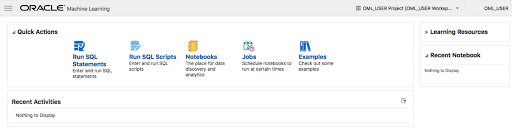Our starting point is to example what is listed in the Examples section. Click on the Examples link. The following lists the example notebooks.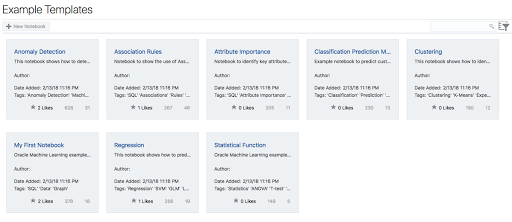Here we have examples that demonstrate how to build Anomaly Detection, Association Rules, Attribute Importance, Classification, Regression, Clustering and one that contains examples of various statistical function.

Click on one of these to see the notebook. The following is the notebook demoing the Statistical Functions. When you select a notebook it might take a few seconds to setup and open. There is some setup needed in the background and to make sure you have access to the demo data and then runs the notebook, generating the results. Most of the demo data is based on the SH schema.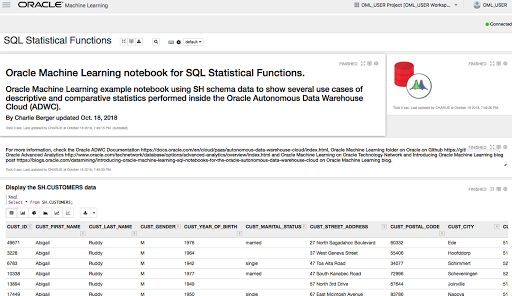Now let us create our first notebook.

From the screen shown above lift on the menu icon on the top left of the screen.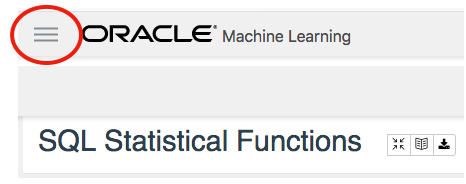And then click on Notebooks from the pop-out menu.In the Notebooks screen click on the Create button to create your first notebook.And give it a meaningful name.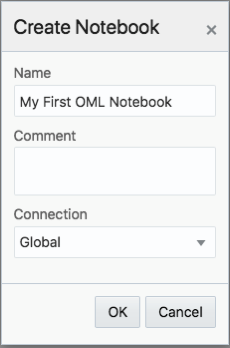The Notebook shell will be created and then opened for you.

In the grey box, just under the name the name of your Notebook, is where you can enter your first SQL statement. Then over on the right hand side of this Cell you will see a triangle on its side. This is the run button.For now you can only run SQL statements, but you also have other notebooks features such as different charting options and these are listed under the grey cell, where your SQL is located.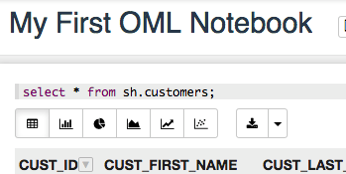Here you can create Bar, Pie, Area, Line and Scatter charts. Here is an example of a Bar chart.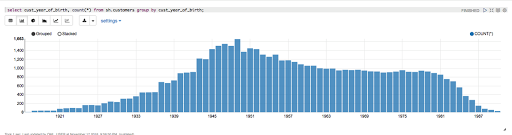Warning: You do need to be careful of your syntax, as minimal details are given on what is wrong with your code. Not even the error numbers.

Go give it a good and see how far you can take these OML Notebooks.

## Wednesday, November 14, 2018

### Reading Data from Oracle Table into Python Pandas - How long & Different arraysize

Here are some results from a little testing I recent did on extracting data from an Oracle database and what effect the arraysize makes and which method might be the quickest.

The arraysize determines how many records will be retrieved in each each batch. When a query is issued to the database, the results are returned to the calling programme in batches of a certain size. Depending on the nature of the application and the number of records being retrieved, will determine the arraysize value. The value of this can have a dramatic effect on your query and application response times. Sometimes a small value works very well but sometimes you might need a larger value.

My test involved using an Oracle Database Cloud instance, using Python and the following values for the arraysize.

`arraysize = (5, 50, 500, 1000, 2000, 3000, 4000, 5000) `

The first test was to see what effect these arraysizes have on retrieving all the data from a table. The in question has 73,668 records. So not a large table. The test loops through this list of values and fetches all the data, using the fetchall function (part of cx_Oracle), and then displays the time taken to retrieve the results.

```# import the Oracle Python library
import cx_Oracle
import datetime
import pandas as pd
import numpy as np

# setting display width for outputs in PyCharm
desired_width = 280
pd.set_option('display.width', desired_width)
np.set_printoptions(linewidth=desired_width)
pd.set_option('display.max_columns',30)

# define the login details
p_host = "************"
p_service = "************"
p_port = "1521"

print('--------------------------------------------------------------------------')
print(' Testing the time to extract data from an Oracle Database.')
print('    using different approaches.')
print('---')
# create the connection

print('')
print(' Test 1: Extracting data using Cursor for different Array sizes')
print('    Array Size = 5, 50, 500, 1000, 2000, 3000, 4000, 5000')
print('')
print('   Starting test at : ', datetime.datetime.now())

beginTime = datetime.datetime.now()
cur_array_size = (5, 50, 500, 1000, 2000, 3000, 4000, 5000)
sql = 'select * from banking_marketing_data_balance_v'

for size in cur_array_size:
startTime = datetime.datetime.now()
cur = con.cursor()
cur.arraysize = size
results = cur.execute(sql).fetchall()
print('      Time taken : array size = ', size, ' = ', datetime.datetime.now()-startTime, ' seconds,  num of records = ', len(results))
cur.close()

print('')
print('   Test 1: Time take = ', datetime.datetime.now()-beginTime)
print('')
```

And here are the results from this first test.

```Starting test at :  2018-11-14 15:51:15.530002
Time taken : array size =  5  =  0:36:31.855690  seconds,  num of records =  73668
Time taken : array size =  50  =  0:05:32.444967  seconds,  num of records =  73668
Time taken : array size =  500  =  0:00:40.757931  seconds,  num of records =  73668
Time taken : array size =  1000  =  0:00:14.306910  seconds,  num of records =  73668
Time taken : array size =  2000  =  0:00:10.182356  seconds,  num of records =  73668
Time taken : array size =  3000  =  0:00:20.894687  seconds,  num of records =  73668
Time taken : array size =  4000  =  0:00:07.843796  seconds,  num of records =  73668
Time taken : array size =  5000  =  0:00:06.242697  seconds,  num of records =  73668
```

As you can see the variation in the results.

You may get different performance results based on your location, network connectivity and proximity of the database. I was at home (Ireland) using wifi and my database was located somewhere in USA. I ran the rest a number of times and the timings varied by +/- 15%, which is a lot!

When the data is retrieved in this manner you can process the data set in the returned results set. Or what is more traditional you will want to work with the data set as a panda. The next two test look at a couple of methods of querying the data and storing the result sets in a panda.

For these two test, I'll set the arraysize = 3000. Let's see what happens.

For the second test I'll again use the fetchall() function to retrieve the data set. From that I extract the names of the columns and then create a panda combining the results data set and the column names.

```startTime = datetime.datetime.now()
print('   Starting test at : ', startTime)
cur = con.cursor()
cur.arraysize = cur_array_size
results = cur.execute(sql).fetchall()
print('   Fetched ', len(results), ' in ', datetime.datetime.now()-startTime, ' seconds at ', datetime.datetime.now())
startTime2 = datetime.datetime.now()
col_names = []
for i in range(0, len(cur.description)):
col_names.append(cur.description[i])
print('      Fetched data & Created the list of Column names in ', datetime.datetime.now()-startTime, ' seconds at ', datetime.datetime.now())
```

The results from this are.

```      Fetched  73668  in  0:00:07.778850  seconds at  2018-11-14 16:35:07.840910
Fetched data & Created the list of Column names in  0:00:07.779043  seconds at  2018-11-14 16:35:07.841093
Finished creating Dataframe in  0:00:07.975074  seconds at  2018-11-14 16:35:08.037134

Test 2: Total Time take =  0:00:07.975614
```

Now that was quick. Fetching the data set in just over 7.7788 seconds. Creating the column names as fractions of a millisecond, and then the final creation of the panda took approx 0.13 seconds.

For the third these I used the pandas library function called read_sql(). This function takes two inputs. The first is the query to be processed and the second the name of the database connection.

```print(' Test 3: Test timing for read_sql into a dataframe')
cur_array_size = 3000
print('   will use arraysize = ', cur_array_size)
print('')
startTime = datetime.datetime.now()
print('   Starting test at : ', startTime)

df2 = pd.read_sql(sql, con)

print('      Finished creating Dataframe in ', datetime.datetime.now()-startTime, ' seconds at ', datetime.datetime.now())
# close the connection at end of experiments
con.close()
```

and the results from this are.

```   Test 3: Test timing for read_sql into a dataframe will use arraysize =  3000

Starting test at :  2018-11-14 16:35:08.095189
Finished creating Dataframe in  0:02:03.200411  seconds at  2018-11-14 16:37:11.295611
```

You can see that it took just over 2 minutes to create the panda data frame using the read_sql() function, compared to just under 8 seconds using the previous method.

It is important to test the various options for processing your data and find the one that works best in your environment. As with most languages there can be many ways to do the same thing. The challenge is to work out which one you should use.

## Tuesday, November 6, 2018

### Installing and configuring Oracle 18c XE

The following are the simple steps required to install Oracle 18c XE (express edition) on Oracle Linux. Check out my previous blog post on Oracle 18c XE. Also check out the product webpage for more details and updates. There is a very important word on that webpage. That word is 'FREE' and is something you don't see too often. Go get and use the (all most) full enterprise version of the Oracle Database.

I've created a VM using Oracle Linux for the OS.

After setting up the VM, login as root and download the RPM file.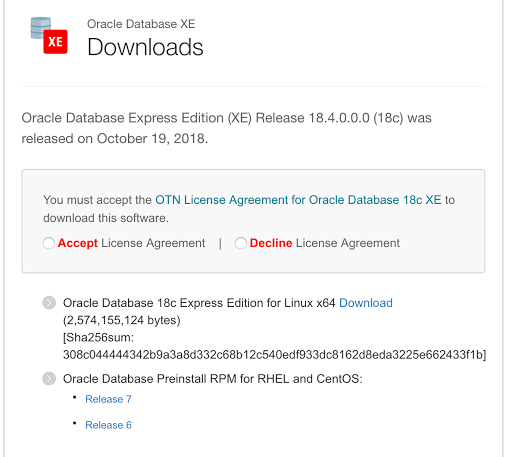Run the following as root to perform dependency checks and configurations.

`yum install -y oracle-database-preinstall-18c `

You can now run the install using the following command.

`yum -y localinstall oracle-database-xe-18c-1.0-1.x86_64.rpm. `

When the install has completed, the next step is to install the database. This is done using the following command.

`/etc/init.d/oracle-xe-18c configure `

You will be prompted to enter a common password for the SYS, SYSTEM and PDBADMIN users. You will need to change these at a later time.

Then to start the database, run

`systemctl start oracle-xe-18c `

The next time you restart the VM, you might find that the database hasn't started or loaded. You will need to do this manually. This is a bit of a pain in the behind.

To avoid having to do this each time, run the following commands as root.

```systemctl daemon-reload
systemctl enable oracle-xe-18c
```

These commands will allow the database to be shutdown when the machine or VM is being shutdown and will automatically start up the database when the machine/VM startups again.

The final step is to connect to the database

`sqlplus sys///localhost:1521/XE as sysdba `

You can then go and perform all your typical admin tasks, set up SQLDeveloper, and create additional users.

Bingo! All it good now.

Docker

Putting Oracle 18c XE on docker is an excellent way to make it easily deployable and to build out solutions that require a DB.

Check out these links for instructions on how to setup a Docker container with Oracle 18c XE.

https://github.com/fuzziebrain/docker-oracle-xe